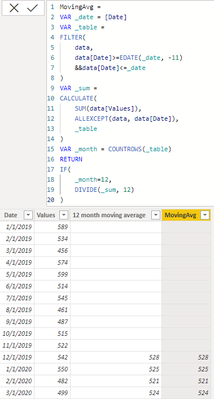cancel
Showing results for
Did you mean:Regular Visitor

## measure rolling 12 month average (returning blank when there are not enough months)

Dear all,

Apologies for this question, I see it is fairly common, but after spending a lot of time trying different methods I still couldn't figure it out completely.

I have a table with values and dates (I also have a date table).  See the table below as sample data. The third colomn is what I am trying to achieve.  I want to calculate a 12 month moving average, where the values are blank if there is not enough data to calculate over the 12 months.  I found this question at this forum the most helpful but still couldn't fully figure it out.

Thanks a lot for your help

 Date Values 12 month moving average jan 2019 589 feb 2019 534 mar 2019 456 apr 2019 574 may 2019 599 jun 2019 514 jul 2019 545 aug 2019 461 sep 2019 487 oct 2019 515 nov 2019 522 dec 2019 542 528 jan 2020 550 524 feb 2020 482 520 mar 2020 499 524

1 ACCEPTED SOLUTIONSuper User

Hi @ae1999
Please refer to attached sample file with the solution``````12 month moving average =
VAR FirstDateWithData = CALCULATE ( MIN ( Sales[Order Date] ), REMOVEFILTERS ( ) )
VAR ReferenceDate = EOMONTH ( FirstDateWithData, 11 )
VAR CurrentDate = MAX ( 'Date'[Date] )
RETURN
IF (
CurrentDate >= ReferenceDate,
CALCULATE (
AVERAGEX ( VALUES ( 'Date'[Year Month] ), [Values] ),
DATESINPERIOD ( 'Date'[Date], CurrentDate, -1, YEAR )
)
)``````
6 REPLIES 6Community Support

Hi, @ae1999

You can try the following methods.

``````12 month moving average =
Var _N1=SUMMARIZE(FILTER('Table',[Date]<=EARLIER('Table'[Date])),[Date],"Sum",SUM('Table'[Values]))
Var _N2=TOPN(12,_N1,[Date],DESC)
Var _Average=DIVIDE(SUMX(_N2,[Sum]),12)
return
IF(COUNTX(_N2,[Sum])<12,BLANK(),_Average)````````````Measure =
Var _N1=SUMMARIZE(FILTER(ALL('Table'),[Date]<=SELECTEDVALUE('Table'[Date])),[Date],"Sum",SUM('Table'[Values]))
Var _N2=TOPN(12,_N1,[Date],DESC)
Var _Average=DIVIDE(SUMX(_N2,[Sum]),12)
return
IF(COUNTX(_N2,[Sum])<12,BLANK(),_Average)``````Is this the result you expect?

Best Regards,

Community Support Team _Charlotte

If this post helps, then please consider Accept it as the solution to help the other members find it more quickly.Super User

Hi @ae1999
Please refer to attached sample file with the solution``````12 month moving average =
VAR FirstDateWithData = CALCULATE ( MIN ( Sales[Order Date] ), REMOVEFILTERS ( ) )
VAR ReferenceDate = EOMONTH ( FirstDateWithData, 11 )
VAR CurrentDate = MAX ( 'Date'[Date] )
RETURN
IF (
CurrentDate >= ReferenceDate,
CALCULATE (
AVERAGEX ( VALUES ( 'Date'[Year Month] ), [Values] ),
DATESINPERIOD ( 'Date'[Date], CurrentDate, -1, YEAR )
)
)``````Regular Visitor

Dear Tamerj1,

This turned out great, thanks a lot!Community Champion

hi @ae1999

Hope "Jan 2019" is just a data format and suppoing your date look like this:

 Date Values 12 month moving average 1/1/2019 589 2/1/2019 534 3/1/2019 456 4/1/2019 574 5/1/2019 599 6/1/2019 514 7/1/2019 545 8/1/2019 461 9/1/2019 487 10/1/2019 515 11/1/2019 522 12/1/2019 542 528 1/1/2020 550 525 2/1/2020 482 521 3/1/2020 499 524

try to add a column like this:

``````MovingAvg =
VAR _date = [Date]
VAR _table =
FILTER(
data,
data[Date]>=EDATE(_date, -11)
&&data[Date]<=_date
)
VAR _sum =
CALCULATE(
SUM(data[Values]),
ALLEXCEPT(data, data[Date]),
_table
)
VAR _month = COUNTROWS(_table)
RETURN
IF(
_month=12,
DIVIDE(_sum, 12)
)``````

it shall work like this:Regular Visitor

Dear @Greg_Deckler ,

Thanks a lot for your reply, this is indeed a better way to create a moving average.

I was wondering how I modify this dax to not return a value/ to return blanks when there are not enough periods to calculate the rolling average, like my example?

To illustrate in your example it should not return a value for the first 2 months since March is the first month we got 3 months of data. I was trying with an if statement but couldn't fully get it working.

Thanks a lot again!Super User

Become an expert!: Enterprise DNA
External Tools: MSHGQM
Latest book!:
Mastering Power BI 2nd EditionDAX is easy, CALCULATE makes DAX hard...